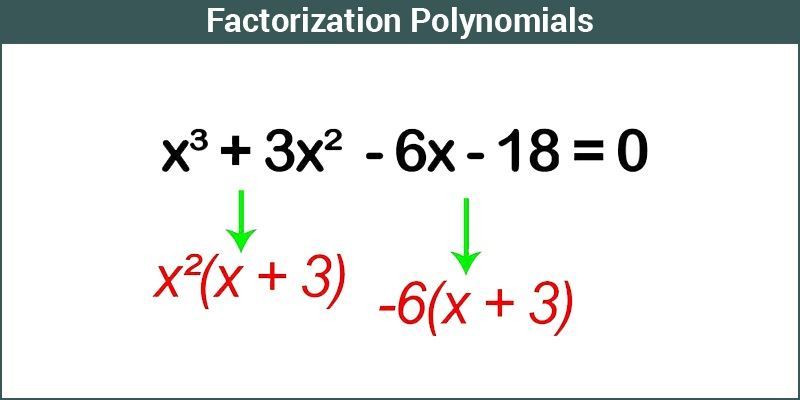# Factorization of Polynomials

A polynomial can be written as the product of its factors having a degree less than or equal to the original polynomial. The process of factoring is called factorization of polynomials.## Prime Factorisation Method

A common technique of factoring numbers is to factor the value into positive prime factors. A prime number is a number whose positive factors are only 1 and itself. 2, 3,5, 7 are all examples of prime numbers.

For example, there are different ways to factories 12.

12 = (2) (6)

12 = (3) (4)

12 = (1/2) (24)

12 = (-2) (-6)

If we factor a number into positive prime factors, there is only one method of doing it.

One has to determine all the terms that were multiplied to obtain the given polynomial. Then try to factor every term that you got in the first step and this continues until you cannot factor further. When you can’t perform any more factoring, it is said that the polynomial is factored completely.

## Factoring Polynomials Types

The different types of factorization of Polynomials are:

• Greatest Common Factor (GCF)
• Grouping
• The difference in Two Squares
• Sum or Difference in Two Cubes

### Greatest Common Factor

Finding the Greatest Common Factor is the basic method for factoring polynomials and it simplifies the problem.

Strategy for finding the Greatest Common Factor (GCF)

1. Factor each term completely
2. Write a product using each factor that is common to all of the terms
3. One each of these factors uses an exponent equal to the smallest exponent that appears on that factor in any of the terms.

To apply this method, look at all the terms and identify whether there is any common factor in all the terms. If any, then factor it out of the polynomial. Also, in this case, we are using only the distributive law in reverse. The distributive law states that

a (b + c) = ab + ac

Notice that every term has an ‘a’ and so you factor it out by distributive law in reverse in this manner,

ab + ac = a (b + c)

### Grouping

The second technique of factoring is called grouping. This method is used when there is no factor common to all the terms of a polynomial, but there will be factors common to some of the terms.

### Difference in Two Squares

A difference in two perfect squares defines that there should be two terms, where the sign between the two terms is a minus sign, and both the two terms contain perfect squares. The result after the factorization of the difference in two perfect square should contain two binomial terms. One binomial term contains the sum of two terms whereas other contains the difference of two terms. We can say that, (a² -b²) = (a+b)(a-b).

### Sum or Difference in Two Cubes

In this technique, as with squares, the difference in two cubes means that there will be two terms and each will contain perfect cubes and the sign between the two terms will be negative. For the sum of two cubes, it contains the plus sign between the perfect cube terms. The formula helps here is:

Sum: (x3+y3) = (x+y) (x² -xy +y²)

Difference: (x3-y3) = (x-y) (x² +xy +y²)

## Factoring Polynomial Examples

Example 1: Factorise x4 – 16

Solution:

The above polynomial can be factorized as:

x4 – 16 = (x² + 4) (x² – 4)

We can still factorize (x2-4). Therefore, the final answer will be;

x4 – 16 = (x² + 4) (x + 2) (x – 2)

Example 2: Factorise 8x4 – 4x³ + 10x².

Solution:

First, you can notice that the common factor is 2 among all the term. Also, note that we can factor x² of every term.

Hence,

8x4– 4x³ + 10x² = 2x²(4x² – 2x + 5)

You can check your factoring by multiplying back the terms to make sure that you get the original polynomial.

For more techniques, register with BYJU’S – The Learning App and download the app to learn with ease.